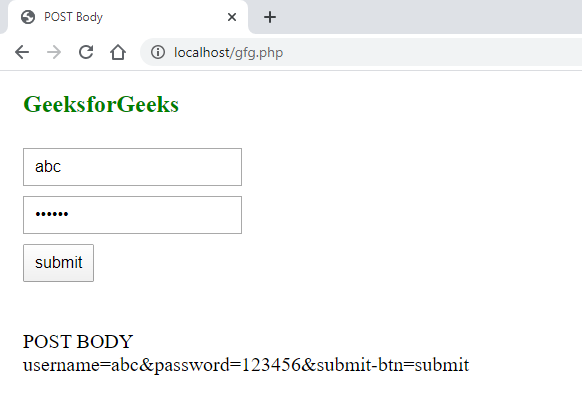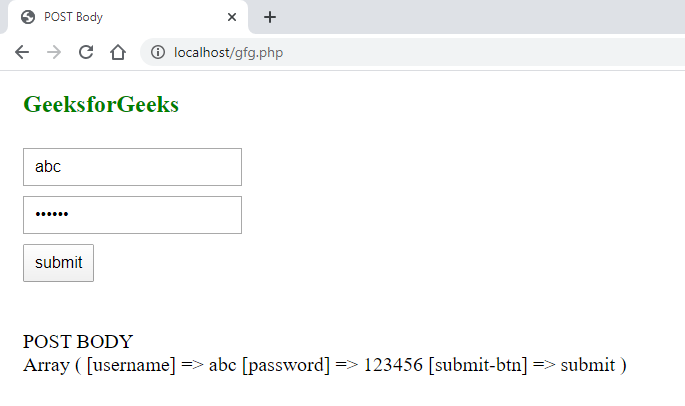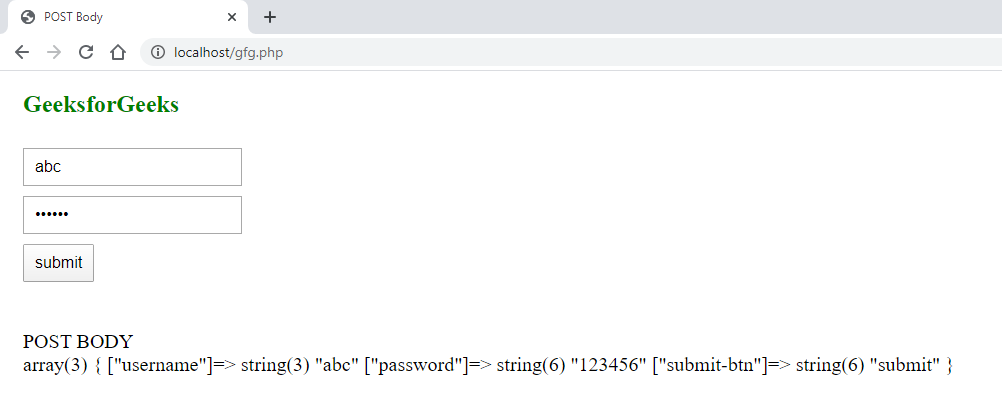GeeksforGeeks App
Open AppBrowser
Continue

# How to get form data using POST method in PHP ?

PHP provides a way to read raw POST data of an HTML Form using php:// which is used for accessing PHP’s input and output streams. In this article, we will use the mentioned way in three different ways. We will use php://input, which is a read-only PHP stream.

We will create a basic HTML form page where we can use all the possible approaches one at a time.
HTML Code:

 ```<``html``>``<``head``>``    ``<``title``>POST Body``    ``<``style``>``        ``form {``            ``margin: 30px 0px;``        ``}``        ``input {``            ``display: block;``            ``margin: 10px 15px;``            ``padding: 8px 10px;``            ``font-size: 16px;``        ``}``        ``div {``            ``font-size: 20px;``            ``margin: 0px 15px;``        ``}``        ``h2 {``            ``color: green;``            ``margin: 20px 15px;``        ``}``    ``````<``body``>``    ``<``h2``>GeeksforGeeks``    ``<``form` `method``=``"post"``>``        ``<``input` `type``=``"text"` `name``=``"username"`  `                           ``placeholder``=``"Enter Username"``>``        ``<``input` `type``=``"password"` `name``=``"password"``                               ``placeholder``=``"Enter Password"``>``        ``<``input` `type``=``"submit"` `name``=``"submit-btn"` `                             ``value``=``"submit"``>``    ````    ``<``br``>`````

Below examples illustrate the approach:
Example 1: In this example, we will use file_get_contents() Function. The file_get_contents() function is used to get the data in string format.

• Syntax:
`file_get_contents('php://input');`
• PHP Code:

 ` POST BODY
"``.``\$post_data``.``""``;        ``    ``}``?>`

• Output:Example 2: In this example, we will use print_r() Function. It is a much simpler way to get the POST data and that is using the print_r() function. This will give the output in the form of an array.

• Syntax:
`print_r(\$_POST);`
• PHP Code:

 ` POST BODY
"``;``        ``print_r(``\$_POST``);``        ``echo` `""``;``    ``}``?>`

• Output:Example 3: We can also use var_dump() function which will give us an array as well but with a bit more information.

• Syntax:
`var_dump(\$_POST);`
• PHP Code:

 ` POST BODY
"``;``        ``var_dump(``\$_POST``);``        ``echo` `""``;``    ``}``?>`

• Output:My Personal Notes arrow_drop_up
Related Tutorials